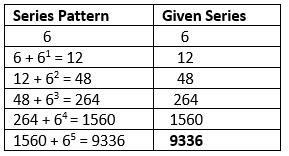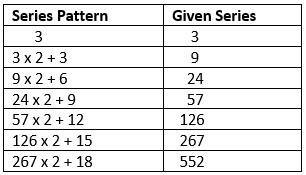# Test: Number Series (Easy)

## 10 Questions MCQ Test Integrated Reasoning for GMAT | Test: Number Series (Easy)

Description
This mock test of Test: Number Series (Easy) for Banking Exams helps you for every Banking Exams entrance exam. This contains 10 Multiple Choice Questions for Banking Exams Test: Number Series (Easy) (mcq) to study with solutions a complete question bank. The solved questions answers in this Test: Number Series (Easy) quiz give you a good mix of easy questions and tough questions. Banking Exams students definitely take this Test: Number Series (Easy) exercise for a better result in the exam. You can find other Test: Number Series (Easy) extra questions, long questions & short questions for Banking Exams on EduRev as well by searching above.
QUESTION: 1

### Look at this series: 4, 6, 12, 30, 90,  ?, 1260. What number should come?

Solution:

Logic is:

► 4 × 1.5 = 6,
► 6 × 2 = 12,
► 12 × 2.5 = 30,
► 30 × 3 = 90,
► 90 × 3.5 = 315,
► 315 × 4 = 1260.

QUESTION: 2

### Look at this series: 6, 12, 48, 264,1560...so on. What number should come next?

Solution:QUESTION: 3

### Look at this series: 7, 10, 8, 11, 9, 12,...so on. What number should come next?

Solution:

This is a simple alternating addition and subtraction series.

In the first pattern, 3 is added. In the second, 2 is subtracted.

► 7 + 3 = 10,
► 10 - 2 = 8,
► 8 + 3 = 11,
► 11 - 2 = 9,
► 9 + 3 = 12,
► 12 - 2 = 10.

QUESTION: 4

Look at this series: 3, 4, 7, 8, 11, 12,...so on. What number should come next?

Solution:

This alternating addition series begins with 3.
► Then 1 is added to give 4,
► Then 3 is added to give 7,
► Then 1 is added to give 8,
► Then 3 is added to give 11,
► Then 1 is added to give 12,
► Then 3 is added to give 15.

QUESTION: 5

Look at this series: 4, 11, 19, 41, ?, 161...so on. What number should come?

Solution:

Pattern:

► 4 × 2 + 3 = 11,
► 11 × 2 – 3 = 19,
► 19 × 2 + 3 = 41,
► 41 × 2 – 3 = 79,
► 79 × 2 + 3 = 161.

QUESTION: 6

Look at this series: 53, 53, 40, 40, 27, 27,...so on. What number should come next?

Solution:

A number is repeated. Then it is reduced by 13 and the result is repeated.

► The next number is 27-13=14.

QUESTION: 7

Look at this series: 8, 22, 8, 28, 8,...so on. What number should come next?

Solution:

This is a simple addition series with a random number, 8, interpolated as every other number.

In the series, 6 is added to each number except 8, to arrive at the next number.

► 22 + 6 = 28,
► 28 + 6 = 34.

QUESTION: 8

Look at this series: 2, 1, (1/2), (1/4),...so on. What number should come next?

Solution:

This is a simple division series, each number is one-half of the previous number.

In other terms to say, the number is divided by 2 successively to get the next result.

► 4/2 = 2
► 2/2 = 1
► 1/2 = 1/2
► (1/2) / 2 = 1/4
► (1/4) / 2 = 1/8 and so on.

QUESTION: 9

Look at this series: 1, 3, 6, 10, 15...so on. What number should come next?

Solution:

It is a triangular number series forming a triangular pattern.

► 1 = 1,
► 1+2 = 3,
► 1+2+3 = 6,
► 1+2+3+4 = 10,
► 1+2+3+4+5 = 15,
► 1+2+3+4+5+6 = 21.

QUESTION: 10

3, 9, 24, 57, ?, 267, 552. What number should come?

Solution: